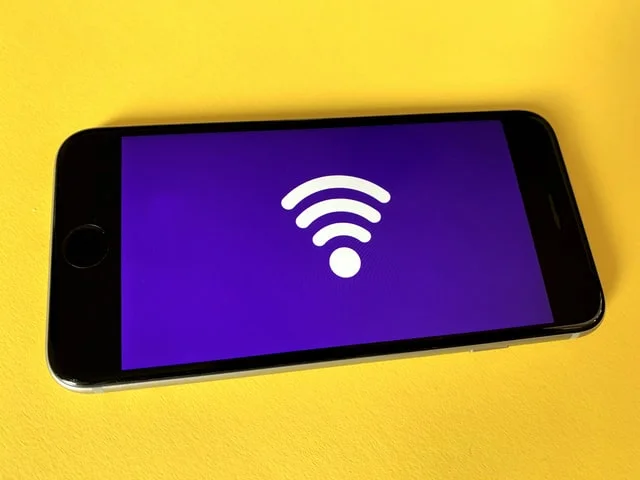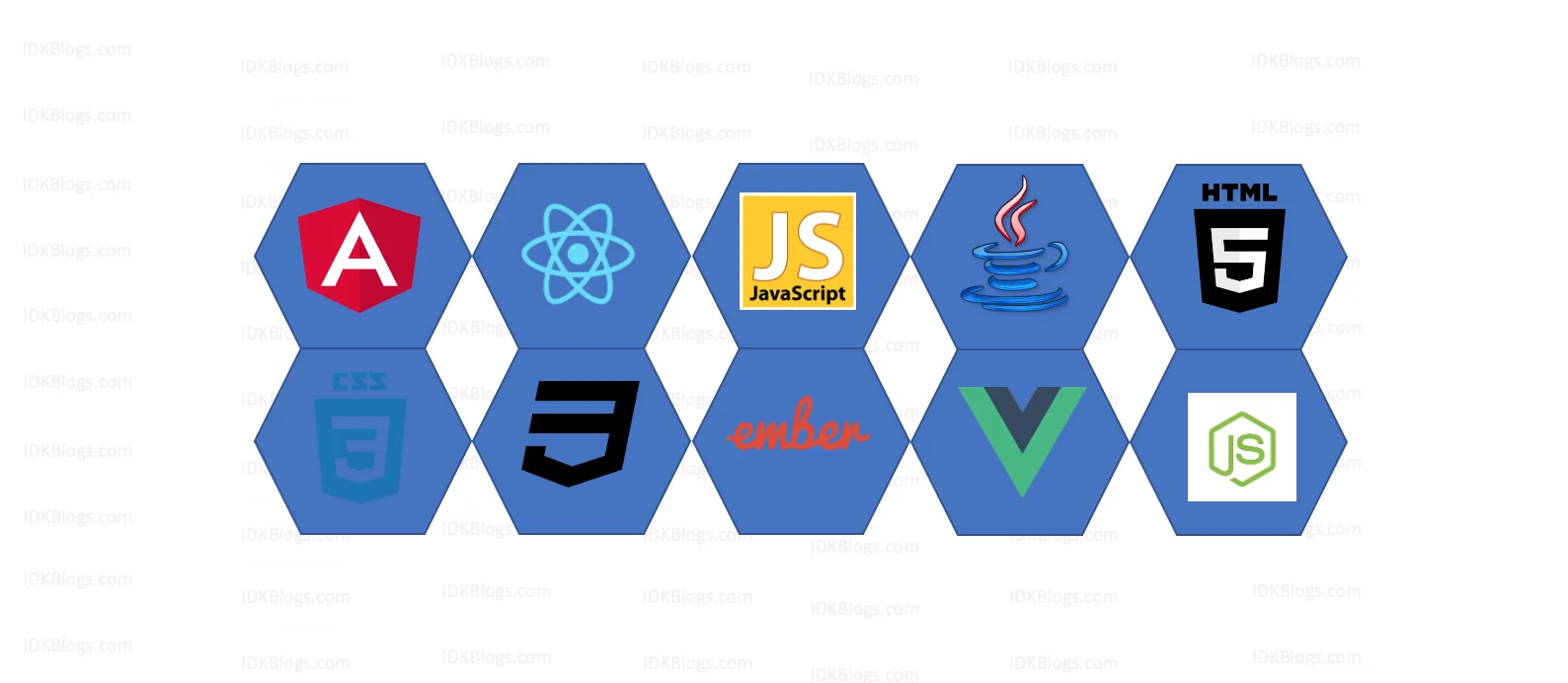# Secure your app data in localStorage

### Let's secure your app's data in localStorage and add another level of security in localStorage.

idkblogs.com      January 9, 2020Shubham VermaA

re you also doing this biggest mistake in web development? Let's not do this in the future.
In the project development, sometimes we need to store our app's data in the localStorage, and just store that data without any security. So this is a security breach of that project.
In this article, we'll learn how we can secure our localStorage while project development.

### Let's get started:

In this article, we'll encrypt our data and store that data in the browser's storage. So if we encrypt that data then no buddy will see that data and because while encryption we'll use our salt and encryption method so it is impossible to decrypt that data. And if someone alters that data in the localstorage then you get to know that some have altered that data, and you can either log out or this handling as per you.
To implement this feature, we'll use an npm module crypto-js.
Let's install this module by command:

``npm install crypto-js --save``

After the successful installation, let's create a utility file and in that file, we'll write our core logic to encrypt and decrypt the data. So let's create a file and write the below codes:

util.js:
``````                        import CryptoJS from 'crypto-js';

export const encryptData = (data, salt) =>
CryptoJS.AES.encrypt(JSON.stringify(data), salt).toString();

export const decryptData = (ciphertext, salt) => {
const bytes = CryptoJS.AES.decrypt(ciphertext, salt);
try {
return JSON.parse(bytes.toString(CryptoJS.enc.Utf8));
}
catch(err){
return null;
}
}

``````

In the above code, we have created two method, encryptData() and decryptData().
Let's see how we can use these methods in our codes:

1. Encrypt the data using encryptData():
We'll use this method to encrypt the data as:

``````                        import {encryptData} from './utils';

const originalData={
name:'IDKBlogs'
}
const salt = process.env.SALT || '6d090796-ecdf-11ea-adc1-0242ac120003';
const encryptedData = encryptData(originalData, salt);
``````

In the above code, the method encryptData() will return the encrypted data like this:
``U2FsdGVkX19H8Wo1WUvd4GbUX5SZp0u5p5/IAwcC0tX3eV1tyHJ+RMuZTDebTwEiiW+BQHjog6KETMv77Az2Nfn1to5qdPgLMYUA8gaC94S1l+Q3ND5tsijh ...``

2. Decrypt the data using decryptData():
We'll use this method to decrypt the data, this method will return the original data.

``````                        import { decryptData } from './utils';

let mkLocalData = localStorage.getItem('mk');
if(!mkLocalData){
// Handle, if there is no data in localStorage, or if someone deleted the localStorage.
}
const salt = process.env.SALT || '6d090796-ecdf-11ea-adc1-0242ac120003';
const originalData = decryptData(mkLocalData, salt);
if(!originalData){
// will executes if someone altered the code in localstorage.
}
``````

In the above codes, by using method encryptData(), we'll get the original data.

Note:
We can store the salt on the server, and we should keep changing very frequently and auto mapped with the codes.Secure your app data in localStorage

### Other examples:

#### String encryption Example:

``````
var CryptoJS = require("crypto-js");

// Encrypt
var ciphertext = CryptoJS.AES.encrypt('my message', 'secret key 123').toString();

// Decrypt
var bytes  = CryptoJS.AES.decrypt(ciphertext, 'secret key 123');
var originalText = bytes.toString(CryptoJS.enc.Utf8);

console.log(originalText); // 'my message'
``````

#### Object encryption Example:

``````                            var CryptoJS = require("crypto-js");

var data = [{id: 1}, {id: 2}]

// Encrypt
var ciphertext = CryptoJS.AES.encrypt(JSON.stringify(data), 'secret key 123').toString();

// Decrypt
var bytes  = CryptoJS.AES.decrypt(ciphertext, 'secret key 123');
var decryptedData = JSON.parse(bytes.toString(CryptoJS.enc.Utf8));

console.log(decryptedData); // [{id: 1}, {id: 2}]
``````

### Conclusion:

In this article, we explored, what is the best way to store our app's data in the localstorage, and how we can secure that data.

Thank you

To read more about the technologies, Please subscribe us, You'll get the monthly newsletter having all the published article of the last month.

### OTHER POSTS